# 8 Some Remarks on Cosmological Models

R. W. Bass, L. Witten

#### DOI

10.34663/9783945561294-13

#### Citation

Bass, R. W. and Witten, L. (2011). Some Remarks on Cosmological Models. In: The Role of Gravitation in Physics: Report from the 1957 Chapel Hill Conference. Berlin: Max-Planck-Gesellschaft zur Förderung der Wissenschaften.

It is unusual to hypothesize that the four-dimensional space-time universe of general relativity is compact (i.e., “finite”). But in such a case several interesting conclusions can be drawn. In the first place, if the mass distribution is assumed to be continuous, so that the metric tensor has no singularities, then the Euler-Poincaré characteristic of the universe must be zero . This implies, for example, that the universe cannot be a four-dimensional sphere. It also implies that a finite universe cannot be simply-connected, in the sense that the first Betti number cannot vanish. This is reminiscent of Professor J.A. Wheeler’s non-simply connected models.)

In the second place, it seems to be generally known that in a finite cosmology there must exist a closed curve in space-time whose tangent vector at every point is time-like. Professor L. Markus has indicated a proof to us. Let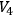denote the 4-manifold of the universe. Now, on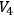construct a continuous, nowhere vanishing field of time-like vectors (, pp. 6-7; cf., p. 207). By Birkhoff’s fundamental theorem on the existence of recurrent orbits in compact dynamical systems , there must exist either an orbit of the type sought or else an “almost-closed” time-like orbit which can serve for the construction of such a closed orbit by an obvious procedure.

A more standard hypothesis, however, is that the universe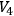is not compact, but is the topological product of the infinite real line (a time axis) with a 3-manifold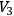. The manifold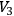is often assumed to be compact, and any local (hence experimentally verifiable) condition which implies compactness is of much interest. For example, ifhas constant curvature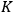then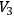is compact if, and only if,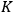is positive (, pp. 84 and 203), and in this case is a 3-sphere if its first Betti number vanishes, and in general admits the 3-sphere as a covering space.

We wish to point out a new method for studying the topology of manifolds such as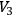and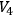. This method consists of the construction of a continuous, nowhere vanishing, irrotational vector field on the manifold under consideration. Once such vector field has been constructed, we can assert that either the manifold is non-compact (i.e., open or “infinite”), or that it cannot be simply-connected.

We shall prove a slight generalization of this theorem; but first, let us note that a similar, but more restrictive and less easily applicable condition is a trivial consequence of Hodge’s well-known theorem that the number of linearly independent harmonic vector fields on a compact Riemannian manifold is equal to its first Betti number. For if after constructing on our manifold an irrotational vector field (which is non-trivial but may vanish at more than one point), we then ascertain that it is also solenoidal (i.e., of vanishing divergence), then the vector field must be harmonic (, p. 56).

Theorem 1 (Hodge):: Let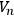be an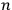-dimensional Riemannian manifold (with positive definite metric tensor), and let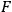denote a non-trivial class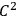vector field defined on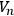. Suppose that the curl and the divergence of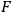both vanish identically; or equivalently, suppose that the fieldsatisfies the generalized Laplace equation for harmonic vector fields. Then, if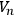is compact, its first Betti number is not zero.

Corollary (Bochner-Myers):: If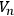is orientable and has positive definite Ricci curvature throughout, then its first Betti number vanishes. (, p. 37).

Recall that the curl tensor of a vector field is independent of the metric tensor, and so is a non-metric notion. Accordingly, the following theorem applies equally well to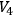with its indefinite hyperbolic metric as to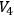with its positive definite Riemannian metric.

Theorem 2:: Let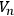be an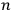-dimensional differentiable manifold, and let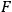be a continuous, class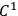vector field defined on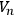. Suppose that F vanishes at most once and that its curl vanishes identically on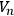. Then eitheris non-compact, or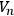is compact and its first Betti number does not vanish. In either case, of course, if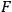actually vanishes nowhere, the Euler-Poincaré characteristic of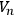is zero.

For non-vanishing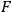this theorem is a consequence of a more general theorem  which applies, for example, to manifolds with boundary. In fact, by a generalization to arbitrary flows of a theorem proved by Lichnerowicz for a very special class of flows (, p. 79), we can prove  that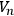is homeomorphic to the product of the real line with an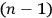-dimensional space which is a connected subset of a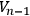. But in the present case, because we are dealing with a manifold, there is a much simpler proof. We wish to thank Professor Kervaire for pointing out to us this simpler proof during the Conference on the Role of Gravitation in Physics. The proof runs as follows. If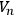is simply connected, then the generalized Stokes Theorem assures us that there exists on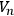a single-valued scalar potential function of which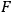is the gradient field. (See the survey of vector analysis in .) But if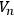is compact, this potential function must assume both its maximum and minimum values on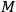, and at these extreme points the gradient must vanish. This contradicts the hypothesis that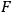has at most one zero on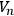, and so proves the theorem.

It is possible that Theorems 1 and 2 have applications to the study of specific cosmological models. In fact, there are many ways of constructing on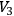, or on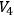continuous vector fields which are unique once the indefinite metric (or set of gravitational potentials) for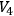has been specified.

Professor J.A. Wheeler has pointed out to us an application of Theorem 2 to.

Theorem 3:: Consider the combined Einstein-Maxwell field theory on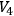. If the vector field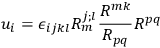is defined everywhere and of class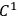on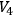, and if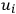does not vanish more than once, then the universecannot be compact.

The vector field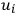, which was defined by Dr. C. W. Misner, is essentially the gradient of the ratio (in a certain coordinate system) of the electric to the magnetic field strength. Dr. Misner has shown that Maxwell’s equations imply that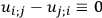. Hence, Theorem 3 follows from Theorem 2.